NEET  >  Test: Physics - 6

# Test: Physics - 6

Test Description

## 45 Questions MCQ Test NEET Mock Test Series | Test: Physics - 6

Test: Physics - 6 for NEET 2023 is part of NEET Mock Test Series preparation. The Test: Physics - 6 questions and answers have been prepared according to the NEET exam syllabus.The Test: Physics - 6 MCQs are made for NEET 2023 Exam. Find important definitions, questions, notes, meanings, examples, exercises, MCQs and online tests for Test: Physics - 6 below.
Solutions of Test: Physics - 6 questions in English are available as part of our NEET Mock Test Series for NEET & Test: Physics - 6 solutions in Hindi for NEET Mock Test Series course. Download more important topics, notes, lectures and mock test series for NEET Exam by signing up for free. Attempt Test: Physics - 6 | 45 questions in 45 minutes | Mock test for NEET preparation | Free important questions MCQ to study NEET Mock Test Series for NEET Exam | Download free PDF with solutions
 1 Crore+ students have signed up on EduRev. Have you?
Test: Physics - 6 - Question 1

### An electromagnetic waves going through vacuum is described by,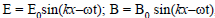, then

Test: Physics - 6 - Question 2

### Because of the space charge in a diode valve,

Test: Physics - 6 - Question 3

### A point source of light is used in a photoelectric effect.If the source is removed farther from the emitting metal,the stopping potential

Test: Physics - 6 - Question 4

A proton and an electron are acceleration by the same potential difference Let λ e and   λ p denote the de Broglie wavelengths of the electron and the proton respectively

Test: Physics - 6 - Question 5

Ionization energy of a hydrogen-like ion A is greater than that of another hydrogen-like ion B. Let r,u,E and L represent the radius of the orbit,speed of the electron, energy of the atom and orbital angular momentum of the electron respectively. In ground state

Test: Physics - 6 - Question 6
The X-ray beam coming from an X-ray tube
Test: Physics - 6 - Question 7
Cutoff wavelength of X-rays coming from a Coolidge tube depends on the
Test: Physics - 6 - Question 8

When an impurity is doped into an intrinsic semiconductor,the cunductivity of the semiconductor

Test: Physics - 6 - Question 9

Two identical p-n junction may be connected in series with a battery in three ways (figure). The potential difference across the two p-n junctions are equal is: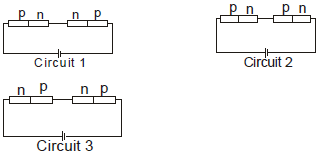Test: Physics - 6 - Question 10
Two identical capacitors A and B are charged to the same potential V and are connected in two circuits at t= 0 as shown in figure. The charges on the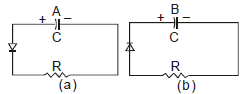capacitors at a time = CR are, respectively,
Test: Physics - 6 - Question 11
In a transistor
Test: Physics - 6 - Question 12
A semiconducting device is connected in a series circuit with a battery and a resistance. A current is found to pass through the circuit. If the polarity of the battery is reversed, the current drops to almost zero. The device may be
Test: Physics - 6 - Question 13

The mass number of a nucleus is

Test: Physics - 6 - Question 14

Two protons are kept at a separation of 10 nm. Let Fn and  F be the nuclear force and the electromagnetic force between them.

Test: Physics - 6 - Question 15

Ten grams of 57Co kept in an open containg beta-decays with a half-life of 270 days. The weight of the material inside the container after 540 days will be very nearly

Test: Physics - 6 - Question 16
A free neutron decays to a proton but a free proton does not decay to a neutron. This is because
Test: Physics - 6 - Question 17
Magnetic field does not cause deflection in
Test: Physics - 6 - Question 18
Beta rays emitted by a radioactive material are
Test: Physics - 6 - Question 19

If elements with principle quantum number n>4 were not allowed in nature, the number of possible elements would be

Test: Physics - 6 - Question 20

Consider the spectral line resulting from the transition n = 2  → n = 1 in the atoms and ions given below. The shortest wave length is produced by

Test: Physics - 6 - Question 21

The equation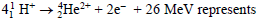Test: Physics - 6 - Question 22
Fast neutrons can easily be slowed down by
Test: Physics - 6 - Question 23

Consider α particles, β particles and γ - rays each having an energy of 0.5 MeV. In increasing order of penetrating powers, the radiations are

Test: Physics - 6 - Question 24

The KX-ray emission line of tungsten occurs at λ  =0.021 nm. The energy difference between K and L levels in this atom is about

Test: Physics - 6 - Question 25
The electrical conductivity of a semiconductor increases when em radiation of wavelength shorter than 2480 nm is incident on it. The band gap (in eV) for the semiconductor is
Test: Physics - 6 - Question 26
The dominant mechanisms for motion of charge carriers in forward and reverse biased silicon p-n junctions are
Test: Physics - 6 - Question 27
As per Bohr model, the minimum energy (in eV) required to remove an electron from the ground state of doubly in ionized Li atom (Z=3) is
Test: Physics - 6 - Question 28
The circuit shown in the figure contains tow diodes each with a forward resistance of 50 ohms and with infinite backward resistance. If the battery voltage is 6V, the current through the 100 ohm resistance (inAmperes) is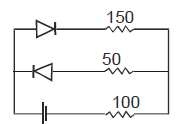Test: Physics - 6 - Question 29
Which of the following statement is not ture ?
Test: Physics - 6 - Question 30

A particle of mass M at rest decays into two particles of masses m1 and m2, having nonzero velocities. The ratio of the de Broglie wavelength of the particles λ 1 / λ 2 is

Test: Physics - 6 - Question 31

Binding energy per nucleon vs. mass number curve for nuclei is shown in the figure W,X,Y and Z are four nuclei indicated on the curve. The process that would release energy is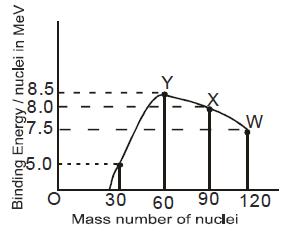Test: Physics - 6 - Question 32

The transition from the state n = 4 to n =3 in a hydrogen-like atom results in ultraviolet radiation. Infrared radiation will be obtained in the transition

Test: Physics - 6 - Question 33

A Hydrogen atom and a Li ++ ion are in the second excited state. If lH and  l Li are their respectively electronic angular momenta, and EH and E Li their respectively enrgies, then

Test: Physics - 6 - Question 34

In a photoelectric experiment anode potenial is plotted against plate current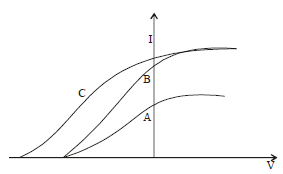Test: Physics - 6 - Question 35

If a star can convert all the He nuclei completely into oxygen nuclei. The energy released per oxygen nuclei is [Mass of He nucleus is 4.0026 amu and mass of Oxygen nucleus is 15.9994]

Test: Physics - 6 - Question 36
The largest wavelength in the ultraviolet region of the hydrogen spectrum is 122 nm. The smallest wavelength in the infrared region of the hydrogen spectrum (to the nearrest integer) is
Test: Physics - 6 - Question 37

Direction : Read the following question and choose
A. If both assertion and reason are true and reason is the correct explantion of assertion
B. If both assertion and reason are true but reason is not the correct explanation of assertion
C. If assertion is true but reason is false
D. If assertion is false but reason is true
Assertion : Nuclear density is almost same for all nuclei
Reason: Size of nucleus  ∞ A 1/3

Test: Physics - 6 - Question 38
Direction : Read the following question and choose
A. If both assertion and reason are true and reason is the correct explantion of assertion
B. If both assertion and reason are true but reason is not the correct explanation of assertion
C. If assertion is true but reason is false
D. If assertion is false but reason is true
Assertion: A free neutron is not a stable particle
Reason: It decays spontaneously into a proton, an electron and an antineutrino
Test: Physics - 6 - Question 39
Direction : Read the following question and choose
A. If both assertion and reason are true and reason is the correct explantion of assertion
B. If both assertion and reason are true but reason is not the correct explanation of assertion
C. If assertion is true but reason is false
D. If assertion is false but reason is true
Assertion: In outermost stationary orbit, energy of electron is least negative
Reason: In such an orbit, electron is at maximum distance from the nucleus
Test: Physics - 6 - Question 40
Direction : Read the following question and choose
A. If both assertion and reason are true and reason is the correct explantion of assertion
B. If both assertion and reason are true but reason is not the correct explanation of assertion
C. If assertion is true but reason is false
D. If assertion is false but reason is true
Assertion: On alpha-decay, group of daughter nucleus shifts
two places to the left from the group of parent nucleus
Reason : An alpha particle carries four units of mass
Test: Physics - 6 - Question 41
Direction : Read the following question and choose
A. If both assertion and reason are true and reason is the correct explantion of assertion
B. If both assertion and reason are true but reason is not the correct explanation of assertion
C. If assertion is true but reason is false
D. If assertion is false but reason is true
Assertion: Balmer series lies in the visible region of electromagnetic spectrum
Reasion :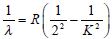where K = 3,4,5....
Test: Physics - 6 - Question 42
Direction : Read the following question and choose
A. If both assertion and reason are true and reason is the correct explantion of assertion
B. If both assertion and reason are true but reason is not the correct explanation of assertion
C. If assertion is true but reason is false
D. If assertion is false but reason is true
Assertion : X-rays travel with the speed of light
Reason : X-rays are electromagnetic rays
Test: Physics - 6 - Question 43
Direction : Read the following question and choose
A. If both assertion and reason are true and reason is the correct explantion of assertion
B. If both assertion and reason are true but reason is not the correct explanation of assertion
C. If assertion is true but reason is false
D. If assertion is false but reason is true
Assertion : The conductivity of intrinsic semiconductors at 0 K is zero
Reason: At 0 K no energy is available. No covalent bond can be broken. No free electron is available for conduction
Test: Physics - 6 - Question 44
Direction : Read the following question and choose
A. If both assertion and reason are true and reason is the correct explantion of assertion
B. If both assertion and reason are true but reason is not the correct explanation of assertion
C. If assertion is true but reason is false
D. If assertion is false but reason is true
Assertion: When base region has larger width, the collector current increases
Reason: Electron hole combination in base results in increase of base current
Test: Physics - 6 - Question 45
Direction : Read the following question and choose
A. If both assertion and reason are true and reason is the correct explantion of assertion
B. If both assertion and reason are true but reason is not the correct explanation of assertion
C. If assertion is true but reason is false
D. If assertion is false but reason is true
Assertion: A hole is a seat to positive charge
Reason: Hole is created when an electron breaks away from a covalent bond in a semiconductor

## NEET Mock Test Series

1 videos|25 docs|144 tests
 Use Code STAYHOME200 and get INR 200 additional OFF Use Coupon Code
Information about Test: Physics - 6 Page
In this test you can find the Exam questions for Test: Physics - 6 solved & explained in the simplest way possible. Besides giving Questions and answers for Test: Physics - 6, EduRev gives you an ample number of Online tests for practice

## NEET Mock Test Series

1 videos|25 docs|144 tests

### How to Prepare for NEET

Read our guide to prepare for NEET which is created by Toppers & the best Teachers